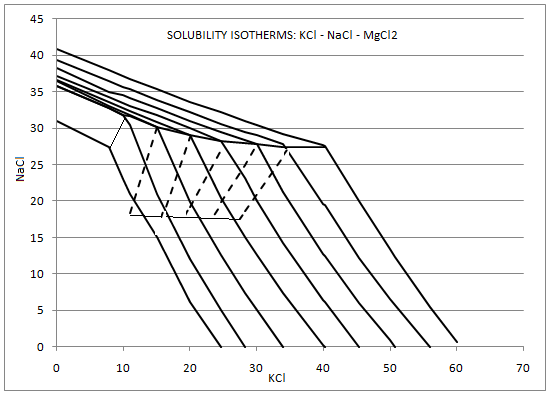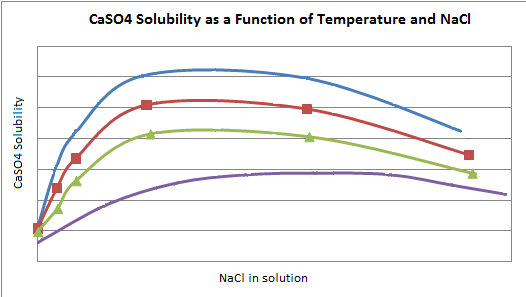# Potash Solubility

Navigation: Models ➔ Potash Models ➔ Potash Species Model ➔ Potash Solubility

Related Links: Species Models, Evaluation Blocks, Solubility, Plant Model - Soluble Tab

## Overview

The solubility of KCl in Potash facilities is a function of temperature and is also influenced by the presence of other species in solution, most importantly NaCl and MgCl2. The curve for KCl solubility as a single species is not valid when other species are present, in this case the saturated value for KCl is lower than the saturated for the solution where only KCl is present.

Similarly, the saturated value for NaCl is lower in solutions that contain KCl and other species.

MgCl2 is often present in reasonably high concentrations in potash solutions, so this has a noticeable effect on the saturated values of KCl and NaCl. Other species that may also affect the saturation values are CaSO4, CaCl2 and LiCl. SysCAD has functionality that allows these species to be included in the calculation of KCl and NaCl solubilities.

Therefore, tables of saturated values for KCl and NaCl where other species are present have been included in SysCAD and are available in the Potash add-on.

SysCAD will automatically calculate the Heat of Reaction (HOR) for the dissolution or precipitation reactions for KCl and NaCl based on the thermodynamic data in the Species database for these species.

• Users must have the Potash Add-on to use the Potash Solubility.
• Potash Solubility can not be enabled Globally in Build139 or later.
• Selectively applying solubility in unit models - The user may selectively enable solubility in units with Evaluation Blocks (EB) such as ties, tanks, pipes, etc.
• Solubility Display in Units - Information specific to the Potash Solubility is described in this section. The general solubility information shown in the access window of pipes, tanks, etc. is described in General Solubility Information in Units and Pipes.
• Enabling High MgCl2 region - 12 - 38g/100g - The region of low MgCl2 concentration, 0 to 12g/100g water, is based on data from literature1. The high MgCl2 concentration region has been interpolated from a small number of scattered data points. This region can be enabled in the Plant Model, but must be used with caution.
• The PHREEQC data set has been added in Build 139, user can select this data set from the Plant Model - Soluble Tab. For this DataSet, the maximum MgCl2 effect is 35g/100g. The imposed maximum is so that the entire solubility system is in a region where MgCl2 is below the saturation point.

## Theory

### KCl - NaCl Solubility

The dissolution of the following speices produces ions in solution:

$\ce{ KCl }$$\ce{ K+ }$ and $\ce{ Cl- }$;
$\ce{ NaCl }$$\ce{ Na+ }$ and $\ce{ Cl- }$;
$\ce{ MgCl2 }$$\ce{ Mg^2+ }$ and $\ce{ 2 Cl- }$.
$\ce{ CaSO4 }$$\ce{ Ca^2+ }$ and $\ce{ SO4^2- }$;
$\ce{ CaCl2 }$$\ce{ Ca^2+ }$ and $\ce{ 2 Cl- }$;
$\ce{ LiCl }$$\ce{ Li+ }$ and $\ce{ Cl- }$.

Notes:

1. In SysCAD we represent these ions as the species, for instance KCl(aq), NaCl(aq) and MgCl2(aq), with the understanding that these species do not actually exist as species, but rather as ions.
2. If more than a single species exists in solution, the solubility of each will be affected by the other due to the interactions between the ions.
• This interaction can be described using the common ion effect at low concentrations of the species.
• However, as the concentrations of the species increase, this theory no longer correctly describes the solubility.
3. The saturated values of KCl(aq) and NaCl(aq) in solutions containing either KCl and NaCl or KCl, NaCl have been obtained from values available in published data. (references 1 and 2).
4. If any of the other optional species, MgCl2, CaSO4, CaCl2 or LiCl, are also present in the solution then they will depress the solubility of the KCl and NaCl. In SysCAD these other species are all combined into a single value and represented as 'MgCl2 Equivalent'. This value is then used to determine the depression of the solubility of the KCl and NaCl.
5. The data is usually displayed in a graph with the form as shown below (ref. 1):### MgCl2 Equivalent

The presence of other species in solution will depress the solubility, and hence the saturation, values of KCl and NaCl. To determine the amount of depression, all of the species may be combined into a single 'MgCl2 Equivalent' value using the following formula:

MgCl2 Equivalent = MgCl2 + a*CaCl2 + b*CaSO4 + c*LiCl

where a, b and c are user defined factors that are set in the Plant Model access window.


The single MgCl2 Equivalent value is then used to determine the depression of solubility of the KCl and NaCl.

Notes:

1. These species are all optional and will only be used by SysCAD if they exist in the project.
2. The user defines the values of a, b and c on the Potash tab of the Plant Model access window. These constants can only be defined for species that exist in the actual project. For example, if the project configuration does not contain LiCl, then the field for LiCl will not be visible.
3. The user may elect to ignore the effect of any of these species by either:

### CaSO4 Solubility

The aqueous form of calcium sulphate, CaSO4(aq), is often present in solutions in Potash facilities. The precipitation / dissolution reaction is:

CaSO4(aq) + H2O(l) <=> CaSO4.2H2O(s)

The solubility of CaSO4(aq) is also affected by the presence of other ions in solution. In the Potash Solubility calculations the user may choose to determine the solubility of CaSO4(aq) in solution using either of the following methods:

1. Use solubility data from Perry (ref. 3) - therefore ignore any other species in solution and use data for 'pure' CaSO4; or
2. Use solubility data from d'Ans (Ref. 1), which includes the effect of NaCl in solution. See the graph below:### Assumptions

1. MgCl2 is NEVER saturated. The presence of this species affects the saturation values of KCl and NaCl, but it does not reach saturation. The MgCl2 saturation value is obtained from the data in Perry. Carnallite is never formed.
2. The solubility data for D'Ans DataSet for KCl and NaCl is valid for values of MgCl2 (or equivalent) to a maximum value of 12g/100g water.
3. For D'Ans Data for KCl and NaCl solubility above 12g/100g MgCl2 has been interpolated from the low MgCl2 region and a small amount of data for this region. The values in the high MgCl2 region MUST be used with caution.
4. The solubility data for PHREEQC DataSet for KCl and NaCl is valid for values of MgCl2 (or equivalent) to a maximum value of 35g/100g water.